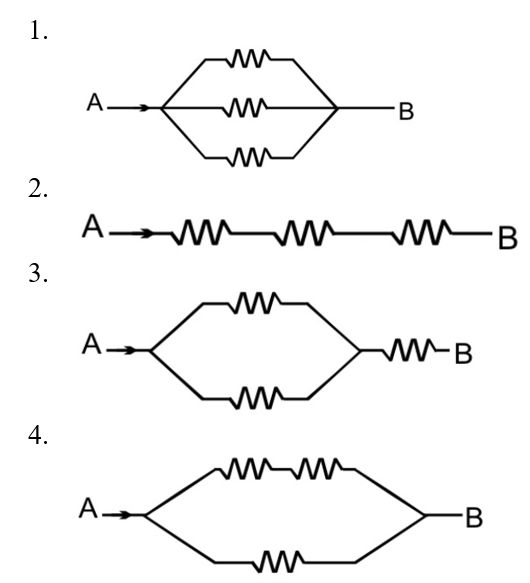Arrange the order of power dissipated in the given circuits, if the same current is passing through all circuit and each resistor is ‘r’:

# Arrange the order of power dissipated in the given circuits, if the same current is passing through all circuit and each resistor is ‘r’:1. A

${\mathrm{P}}_{2}>{\mathrm{P}}_{3}>{\mathrm{P}}_{4}>{\mathrm{P}}_{1}$

2. B

${\mathrm{P}}_{3}>{\mathrm{P}}_{2}>{\mathrm{P}}_{4}>{\mathrm{P}}_{1}$

3. C

${\mathrm{P}}_{4}>{\mathrm{P}}_{3}>{\mathrm{P}}_{2}>{\mathrm{P}}_{1}$

4. D

${\mathrm{P}}_{1}>{\mathrm{P}}_{2}>{\mathrm{P}}_{3}>{\mathrm{P}}_{4}$

Register to Get Free Mock Test and Study Material

+91

Verify OTP Code (required)

### Solution:

$\mathrm{P}={\mathrm{i}}^{2}{\mathrm{R}}_{\mathrm{eq}}$

${\mathrm{R}}_{1}=\frac{\mathrm{R}}{3}$

${\mathrm{R}}_{2}=3\mathrm{R}$

${\mathrm{R}}_{3}=\frac{3\mathrm{R}}{2}$

${\mathrm{R}}_{4}=\frac{2\mathrm{R}}{3}$

${\mathrm{R}}_{2}>{\mathrm{R}}_{3}>{\mathrm{R}}_{4}>{\mathrm{R}}_{1}$

Thus, ${\mathrm{P}}_{2}>{\mathrm{P}}_{3}>{\mathrm{P}}_{4}>{\mathrm{P}}_{1}$Register to Get Free Mock Test and Study Material

+91

Verify OTP Code (required)